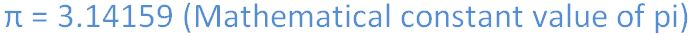# PI number (PI) in JavaScript

#### PI Property

The number `PI` (π) is a mathematical constant, the ratio of a circle cirumference to its diameter. It is represented by the Greek letter "π" since the 18th century and spelled as '`pi`' some times. `PI` (π) is an irrational number and it cannot be expressed exactly as a fraction.The `PI` property returns the ratio of a circle's area to the square of its radius, which is approximately 3.14159.

```<p>Click the below button to return PI.</p>
<input type="button" onclick="myNumber()" value="Click">
<p id="myId"></p>

<script>
function myNumber() {
document.getElementById("myId").innerHTML = Math.PI;
}
</script>```

In the above code snippet we have given `Id` as "`myId`"to the second `<p>` element in the HTML code. There is a function myNumber() in the`<script>`block which is connected to the onclick of the HTML button. We need to return the ratio of a circle's area to the square of its radius, for that we are using `PI`property. Onclick of the button "Click" in the HTML code fires the function myNumber() in the `<script>` block, at the same time `Math.PI` property returns the ratio of circle's area to the square of its radius as output.

OUTPUTViews: 3347 | Post Order: 93